# High School Math : Using Natural Log and Log-Base-10

## Example Questions

### Example Question #1 : Logarithms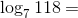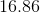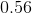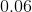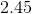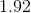Explanation:

Most of us don't know what the exponent would be if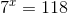and unfortunately there is no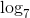on a graphing calculator -- only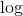(which stands for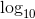).

Fortunately we can use the base change rule: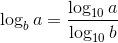Plug in our given values.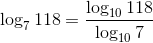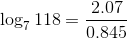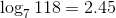### Example Question #1 : Log Base 10

Based on the definition of logarithms, what is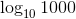?

3

10

4

2

100

3

Explanation:

For any equation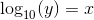,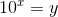. Thus, we are trying to determine what power of 10 is 1000.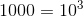, so our answer is 3.

### Example Question #2 : Logarithms

What is the value ofthat satisfies the equation?Explanation:is equivalent to. In this case, you know the value of(the argument of a logarithmic equation) and b (the answer to the logarithmic equation). You must find a solution for the base.